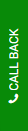# FNCE40002 Data Preparation and Regression Analysis Assessment 1 AnswerAdvanced Investments (FNCE40002) Assignment 1 - Semester 1 2021

 Type: Individual assignment Word limit: 1500 words Marks: This assignment counts 15% towards your final mark in this subject. Precision: Report all estimation results with four digits after the decimal point. Presentation: Use either Times New Roman 12pt or Arial 11pt. For paragraphs, use either double spacing or 1½ spacing. Software: Use a software of your choice for the data preparation and regression analysis. Please do not attach any programming code.

ASSIGNMENT

The first assignment in Advanced Investments reviews your knowledge of the CAPM. In addition, you will become more familiar with regression analysis. The starting point for this assignment is the table from the Fu and Yang (2011) paper on page 26 of the Week 2 slides. Your analysis will complement their results.

Collect the data

Instead of individual stocks, use the “25 Portfolios Formed on Size and Book-to-Market (5x5)” from the online data library of Professor Kenneth R French (use a search machine to locate it). You will also need the “Fama/French 3 Factors” file from the same source. Download the data in a file format that is suitable for your software of choice. Prepare the data as follows:

• Only keep the following information from the first file (scroll down to find these):
• Average Equal Weighted Returns – Monthly from 1926.07 – 2021.01
• Average Market Cap – Monthly from 1926.07 – 2021.01
• Only keep the following information from the second file:

oMonthly from 1926.07 – 2021.01

• Divide all returns and excess returns by 100. Change all net returns to gross returns (careful, do not add a one to the market excess return in the second file).
• Combine all data in a single file (first column should be the date).

CAPM regressions and annualization

Now create a data matrix with 89 rows and 101 columns for the 25 portfolios in the following way (see the notes below for some help; use a loop if possible):

• Use 1926.07 – 1931.06 data to estimate CAPM betas and idiosyncratic volatilities.
• Use 1931.07 – 1932.06 data to obtain annual market and portfolio excess returns.
• Use 1931.07 data to obtain the average market size for the 25 portfolios.
• Save in row 1 of data: 26 returns, 25 betas, 25 idiosyncratic volas, 25 sizes.
• Then continue with:
• Use 1927.07 – 1932.06 data to estimate CAPM betas and idiosyncratic volatilities.
• Use 1932.07 – 1933.06 data to obtain annual market and portfolio excess returns.
• Use 1932.07 data to obtain the average market size for the 25 portfolios.
• Save in row 2 of data: 26 returns, 25 betas, 25 idiosyncratic volas, 25 sizes.
• Then continue like this until you reach:
• Use 2014.07 – 2019.06 data to estimate CAPM betas and idiosyncratic volatilities.
• Use 2019.07 – 2020.06 data to obtain annual market and portfolio excess returns.
• Use 2019.07 data to obtain the average market size for the 25 portfolios.
• Save in row 89 of data: 26 returns, 25 betas, 25 idiosyncratic volas, 25 sizes.

You now have a data matrix with 89 annual observations of excess returns, estimated betas, estimated idiosyncratic volatilities, and market sizes for the 25 portfolios.  Note that you were using the 60 months preceding each annual return to estimate the CAPM betas and idiosyncratic volatilities. Thus, we can evaluate the out-of-sample  performance of beta.

Notes: Recall that you should use excess returns on the left-hand-side of the CAPM regression. Include an intercept. Use the square root of the estimated residual variance from the CAPM regression as an estimate of idiosyncratic volatility. To obtain an annualized excess return, first compound the risky and risk-free returns and then take the difference of these compounded returns.

Prepare and present a graph like on page 22 of the Week 2 slides for the 25 portfolios based on your annual data set. Interpret the graph.(5 marks)

Fama-MacBeth regressions

Now run the following Fama-MacBeth cross-sectional regressions based on the annual data you created under B). Recall that you need to run T = 89 cross-sectional regressions for the N = 25 portfolios and then report the average of the estimated parameters. Always include an intercept in addition to the following (vola = idiosyncratic volatility):

1. Y = excess return, X = log(size)
2. Y = excess return, X = log(vola)
3. Y = excess return, X = CAPM beta
4. Y = excess return, X = log(size), log(vola)
5. Y = excess return, X = log(size), CAPM beta
6. Y = excess return, X = log(vola), CAPM beta
7. Y = excess return, X = log(size), log(vola), CAPM beta
• Report all Fama-MacBeth estimates, standard errors, and t-statistics in one table. Interpret your estimation results with respect to the following two questions:
• Based on your results, is the size effect a size anomaly?(5 marks)
• How do your results compare with Fu and Yang (2011)?(5 marks)